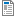National Curriculum

# Year 3/4 Maths Plans

Hamilton provide Mixed Year 3/4 weekly maths plans (below). Y3/4 short blocks will be coming during Summer Term for September 2019. We will be phasing out the plans, as we believe our blocks offer you all of the same advantages and more. Find out more about the timetable for Hamilton's Y3/4 maths blocks.

Supporting documents for set
• Week
• Title
1
+ Details
Number and place value

Year 3: Place two and three-digit numbers on a line; order and compare; understand place value in three-digit numbers; understand and use place value with money. Year 4: Understand what each digit represents in a four-digit number; write place value additions and subtractions; place three-digit numbers on a line; order and place three-digit and four-digit numbers on a line; compare four-digit numbers.Methods used bead bars and landmarked lines.Begin to use written column addition.Autumn Week 1 Plan
2
+ Details

Year 3: Know number bonds to 20 and use for mental addition and subtraction. Use = to represent equality. Add and subtract one-digit to two-digit numbers. Year 4: Add pairs of two-digit numbers and three-digit numbers using place value or counting on. Subtract two-digit numbers from two-digit numbers by counting back or counting up. Methods used include adding near multiples, partitioning, Frog counting up subtraction and counting back subtraction. Includes bar model examples.Autumn Week 2 Plan
3
+ Details
Number and place value

Year 3: Add 2-digit numbers by partitioning; subtract 2-digit numbers and find change from £1 by counting up using Frog. Year 4: Add two or three 3-digit numbers using compact written addition; subtract numbers with 2 or 3 digits by counting up; check subtraction with addition. Methods used include written addition: expanded and compact column addition. Written subtraction: Frog/counting up subtraction, finding change. Includes bar model examples.

4
+ Details
Shape: properties of shapes

Year 3: Recognise lines of symmetry, complete symmetrical drawings; describe, name, sort 2D shapes; describe, name, sort 3D shapes; learn/use correct vocabulary.Year 4: Draw circles with different radii using compasses; describe, name and sort 2D and 3D shapes; draw polygons describe, name and sort triangles; use Venn or Carroll diagrams to sort shapes, discuss properties of shapes.

5
+ Details
Multiplication and division

Year 3: Double 2-digit numbers; halve even numbers; know multiplication and division facts and recognise multiples of 2, 5 and 10 times tables; understand that multiplication is commutative. Year 4: Double and halve two-digit and three-digit numbers using partitioning; know 3, 4, 6, 8 times tables and associated division facts. Includes bar model examples.

6
+ Details
Number, place value and money

Year 3: Understand and use place value to add and subtract (3-digit numbers); use place value in money to add and subtract; add or subtract 1, 10 and 100 to or from 3-digit numbers. Year 4: Use place value to add and subtract 4-digit numbers; add or subtract 1, 10, 100, 1000 to or from 4-digit numbers. Methods used include mental addition and subtraction by partitioning, place value grids and cards, use calculators to show place value.

7
+ Details

Year 3: Use place value to add and subtract 1s, 10s, 100s to and from 2-digit and 3-digit numbers; add and subtract near multiples of 10 from 3-digit numbers. Year 4: Add and subtract 3-digit and 4-digit numbers using place value; add and subtract near multiples from a 3-digit number. Use place value grids and begin to record jottings on empty number lines.

8
+ Details

Year 3: Know pairs of multiples of 5 totalling 100. Know pairs of 2-digit numbers totalling 100. Mental subtraction of numbers on either side of 100 by counting up. Year 4: Written subtraction of 3-digit numbers using decomposition; choose a subtraction calculation strategy. Methods used include expanded decomposition, 'clever counting', Frog/counting up subtraction, recording jottings on empty number lines. Includes bar model examples.

9
+ Details
Time, bar charts and pictograms

Year 3: Tell the time to five minutes using analogue, digital and Roman numeral clocks. Understand and use am/pm times appropriately. Collect data and display using bar charts or pictograms. Year 4: Tell the time to the nearest minute using analogue, digital and Roman numeral clocks. Use am/pm times appropriately. Convert units of time, work out time intervals crossing the hour, collect data and organise into bar charts and pictograms. Methods used include written timelines using Frog to count on.

10
+ Details
Multiplication and division

Year 3: Know multiplication facts for 3 and 4 times tables, derive corresponding division facts. Divide by 2, 3, 4, 5 and 10, including giving remainders. Year 4: Use grid method to multiply a 2-digit number by a 1-digit number. Use chunking on a number line to divide numbers just beyond the tables, leaving no remainders. Methods used for written division include: chunking and for written multiplication: grid method

11
+ Details
Division and fractions

Year 3: Understand concept of a fraction of a shape and quantity. Find fractions of a quantity: ½, ⅓, ¼, ¾, ⅔. Year 4: Divide two-digit numbers by single-digit numbers, just above the 10th multiple with remainders, using written layout. Count in quarters, thirds and tenths saying the equivalent fractions. Find unit fractions and non-unit fraction of amounts. Methods used include empty number lines, chunking and short division. Includes bar model examples.